# 布隆过滤器

## 解决方式

1. 使用MySQL进行存储

这当然是最简单也最直接的办法，但我们知道MySQL的表空间是有上限的，并且在《阿里巴巴JAVA开发规范》中也明确表示：每张表的记录最好不要超过500万。当然，我们也可以进行分表处理，假设每张表存500万，我们需要分出10亿/500万 = 200张表，这显然是不可取的，别说项目经理会把你骂成🐶，你可能也会问自己，这什么JB玩意？

2. BitMap

还记得我们讲位图算法时的问题吗？

假设有2亿个数，范围在0~3亿，给出一个数，判断2亿个数中是否存在该数?

我就问你像不像？

那么我们现在假设使用BitMap解决该问题

初始化操作：定义一个10亿的bit数组，将email进行hash运算，把对应的bit位置为1

判断某个邮箱是否在黑名单内：对该邮箱进行hash，将hash值与bit数组比较，判断对应的bit位是否为1，是则表示这个邮箱是黑名单里的。

时间复杂度：O(1)

空间复杂度：10亿 / 8 / 1024 / 1024 = 119M

好像解决了哈，但是但是，假设我们黑名单上有个email: lzj960515@163.com, 它的hash值为1，这时，有一个不在黑名单的email：lzj960515@gmail.com, 它的hash值也为1, 程序就会出现lzj960515@gmail.com是黑名单上的email的误判。如果这是你的邮箱系统，那你就可能隔三差五收到用户投诉：怎么把我的邮箱屏蔽了？！

那么我们该如何解决这个问题呢？

## 布隆过滤器

### 原理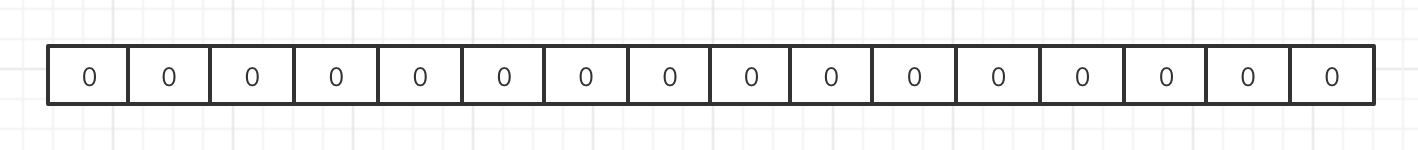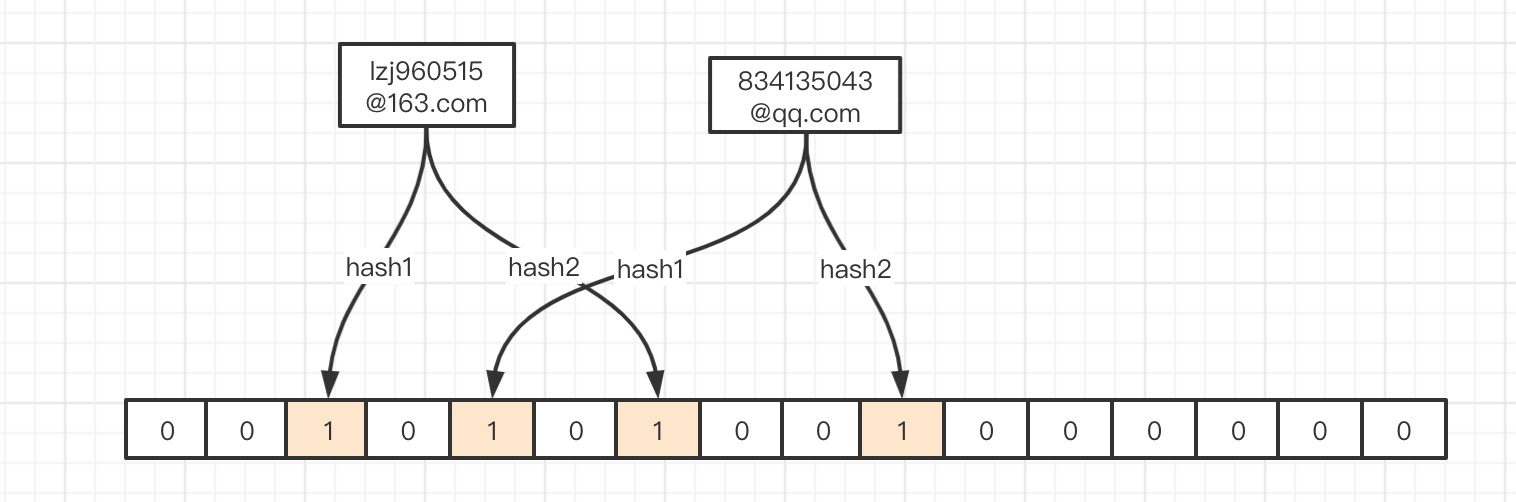hash结果： hash1 -> 6 hash2 -> 11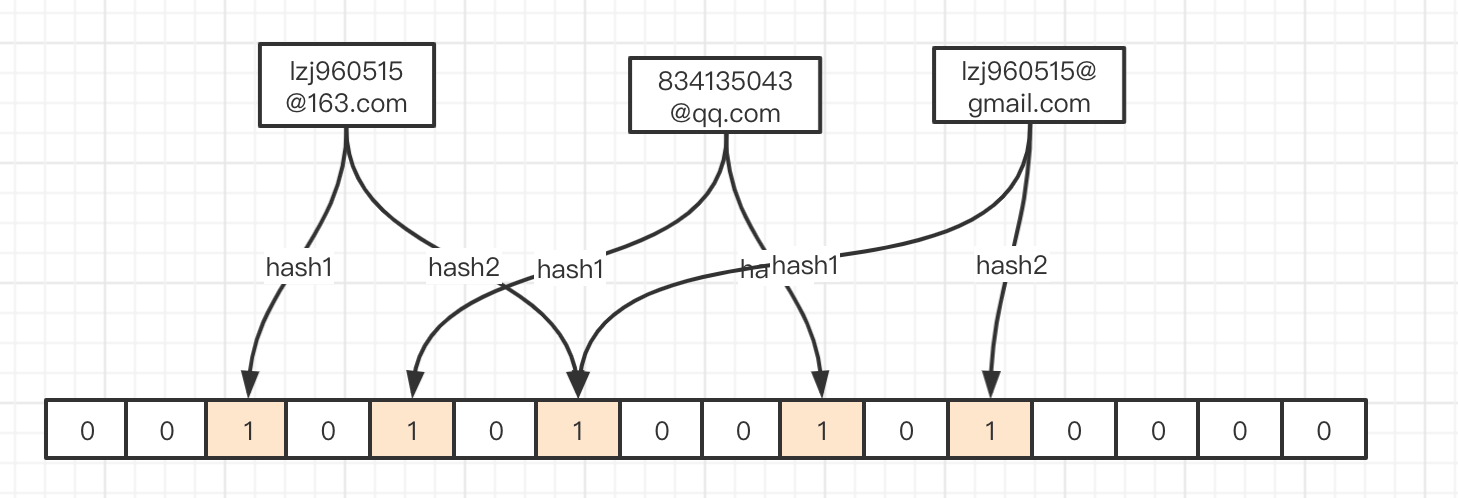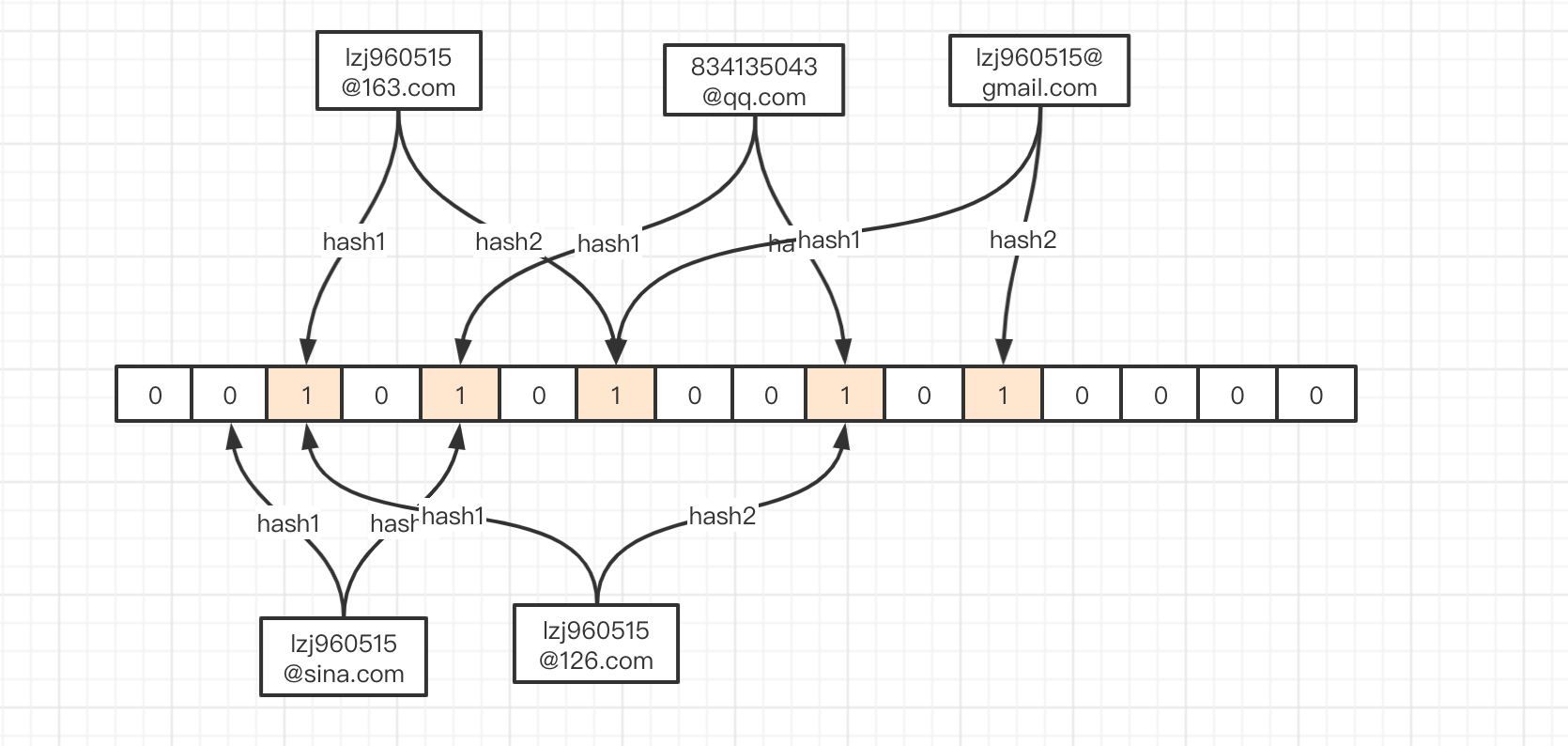hash碰撞只能降低，不能完全解决，可以说有hash的地方就会有hash碰撞

1. 增加数组长度

这一点我想应该是毫无疑问的，当伴随的数组的长度增加，我的hash值落点也自然更加散列，就比如有两个hash值17 、33，当数组长度为16时，他们的落点分别是 17%16=1，33%16=1，此时发生hash碰撞，当数组长度为32时，17%32=17， 33%16=1，hash碰撞消失了。

2. 增加hash函数的数量

在描述上述问题时，我们使用了两个hash函数，如果我们再增加一个hash函数，那么多个hash碰撞的概率就会减小，两次hash你都碰撞了，三次hash总不碰吧，三次还碰，四次呢？当然，我们也不可能无休止的增加下去，这不仅会降低我们的效率，而且一个16bit长度的数组，你搞16个hash函数，玩个蛋！

m为开的长度, n为元素个数, k为哈希函数个数为, p为误判率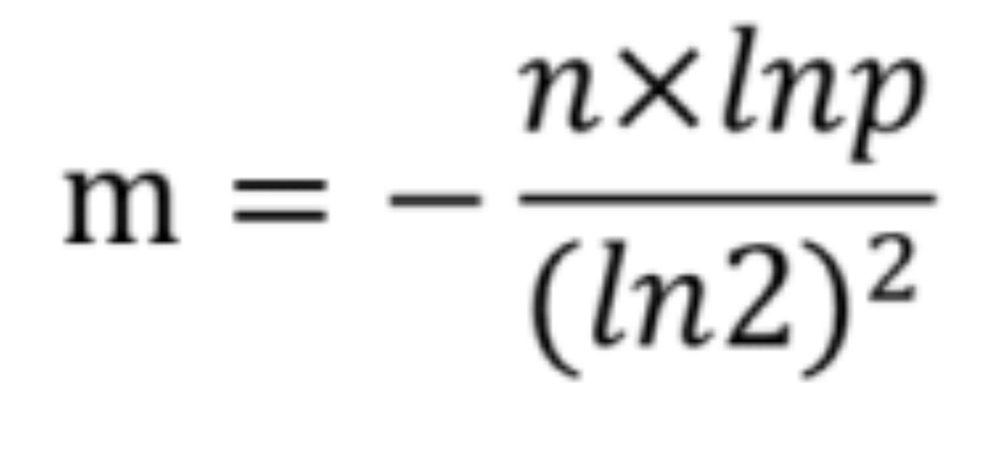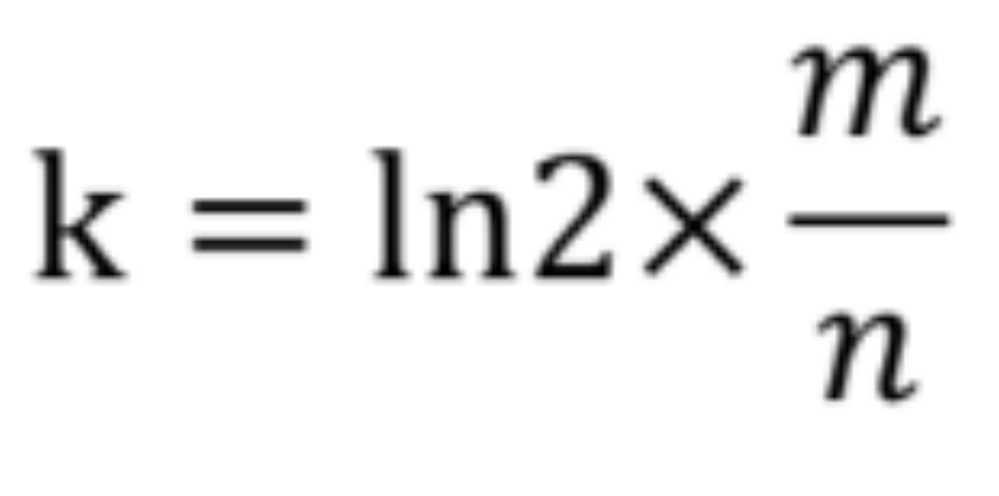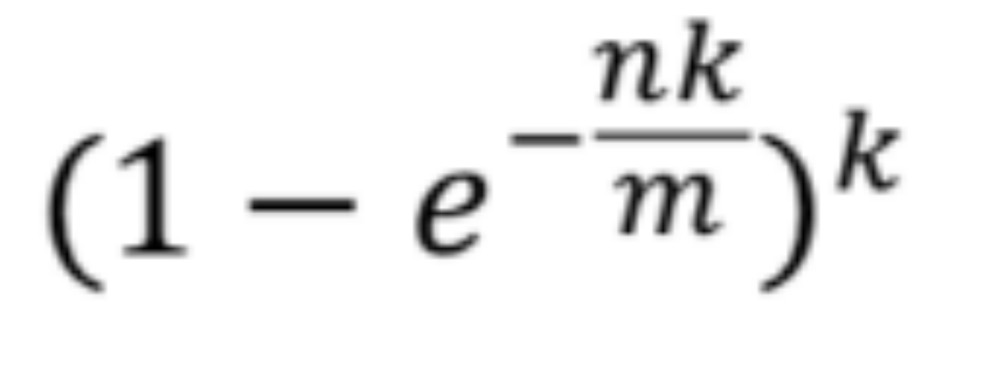### 上代码

``````public class BloomFilter {

int size;
// 这里借用java自带的BitSet
BitSet bits;

public BloomFilter(int size) {
this.size = size;
bits = new BitSet(size);
}

public void add(String key) {	//O(1)
int hash1 = hash_1(key);
int hash2 = hash_2(key);
int hash3 = hash_3(key);

bits.set(hash1, true);
bits.set(hash2, true);
bits.set(hash3, true);
}

public boolean find(String key) {
int hash1 = hash_1(key);
if (!bits.get(hash1))
return false;
int hash2 = hash_2(key);
if (!bits.get(hash2))
return false;
int hash3 = hash_3(key);
if (!bits.get(hash3))
return false;

return true;
}

public int hash_1(String key) {
int hash = 0;
int i;
for (i = 0; i < key.length(); ++i) {
hash = 33 * hash + key.charAt(i);
}
return Math.abs(hash) % size;
}

public int hash_2(String key) {
final int p = 16777619;
int hash = (int) 2166136261L;
for (int i = 0; i < key.length(); i++) {
hash = (hash ^ key.charAt(i)) * p;
}
hash += hash << 13;
hash ^= hash >> 7;
hash += hash << 3;
hash ^= hash >> 17;
hash += hash << 5;
return Math.abs(hash) % size;
}

public int hash_3(String key) {
int hash, i;
for (hash = 0, i = 0; i < key.length(); ++i) {
hash += key.charAt(i);
hash += (hash << 10);
hash ^= (hash >> 6);
}
hash += (hash << 3);
hash ^= (hash >> 11);
hash += (hash << 15);
return Math.abs(hash) % size;
}

public static void main(String[] args) {
// O(1000000000)
//8bit= 1byte
BloomFilter bloomFilter = new BloomFilter(Integer.MAX_VALUE);	//21亿
System.out.println(bloomFilter.hash_1("1"));
System.out.println(bloomFilter.hash_2("1"));
System.out.println(bloomFilter.hash_3("1"));

System.out.println(bloomFilter.find("1"));
System.out.println(bloomFilter.find("1123"));
}
}
``````

``````<dependency>
<artifactId>guava</artifactId>
<version>27.0-jre</version>
</dependency>
``````

``````package cn.zijiancode.pig.util;

/**
* @author Zijian Liao
* @since 1.0.0
*/
public class BloomFilterTest {

public static void main(String[] args) {
//我们要插入的数据也就是n
int datasize = 100000000;
//0.1%	误判率
double fpp = 0.001;

BloomFilter<Integer> bloomFilter = BloomFilter.create(Funnels.integerFunnel(), datasize, fpp);

long start = System.currentTimeMillis();
for(int i = 0 ; i < datasize ; i ++) {
bloomFilter.put(i);
}
System.out.println((System.currentTimeMillis() - start) + ":ms");

// 测试误判率
int count = 0;
for(int i = 200000000 ; i < 300000000 ; i++){
if(bloomFilter.mightContain(i)){
count++;
}
}
System.out.println("误判的个数:" + count);
}
}

``````

``````126902:ms

``````

99777/100000000 = 0.00099777 -> 0.1%

1. 爬虫过滤
2. 缓存击穿
3. 垃圾邮件过滤
4. 秒杀系统中重复购买
5. ...
#